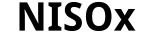## Matlab

A random collection of Matlab m-files and functions to make life easier.

• abline.m
Like R’s abline; useful for quickly addling a line according to `y = a + b * x’, or for putting a horizontal or vertical line on a plot.
• fullpage.m
For printing, make the figure fill up the whole page with small margins.
• minmax.m
Return [min max] for a vector/matrix.
• Median Absolute Deviation Correlation Coefficient, a robust correlation estimator